# Bihar Board 12th Chemistry Important Questions Long Answer Type Part 4 in English

BSEB Bihar Board 12th Chemistry Important Questions Long Answer Type Part 4 are the best resource for students which helps in revision.

## Bihar Board 12th Chemistry Important Questions Long Answer Type Part 4 in English

Question 1.
Describe nucleophilic substitution reaction of haloalkanes.
Nucleophilic substitution reactions in haloalkenes-
The halogen atom in haloalkanes in more electronegative than the carbon atom attached to it. As a result, carbon atom acquires a partial +ve charge and the halogens atom a partial -ve charge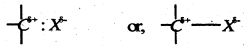The presence of this small +ve charge on the carbon atoms makes it susceptible to attack by nucleophilic reagent (i.e. reagent possessing one pair of electrons). Thus, when a nucleophile stronger than the halide ion approaches the +vely charged carbon atom of an alkyl halide, the halogen atom with its bonding pair of electrons is displaced and a new bond between the carbon atom and the incoming nucleophile is formed.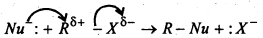Such reactions in which a stronger nucleophilie displaces a weaker nucleophile are called nucleophilic substitution reactions and the atom or group (halide ion) which departs with its bonding pair electrons is called the leaving group. Among the halide ions, the order in which leaving groups depart follows the sequence : I > Br > Cl

Types of nucleophilic substitution reactions- There are two types of nucleophilic substitution reactions.
These are : (i) SN1 (Substitution, nucleophilic unimolecular)
(ii) SN2 (Substitution, nucleophilic biomolecular)
The rate of SN1 reactions depends only upon the concentration of the alkyl halide and is independent of the concentration of nucleophilic, i.e. Rate = K[RX]
The rate of SN2 reaction depends upon the concentration of both the alkyl halide as well as nucleophile.
i.e. Rate = K [RX] [Nu:]
All primary alkyl halide undergo substitution by SN2 mechanism while secondary alkyl halides may undergo substitution either by SN2 or SN1 mechanism depending upon the reaction conditions. But all tertiary halides under to substitution by SN1 mechanism.

(i) Substitution of helogen by hydroxyl group- Haloalkanes on treatment with boiling aqueous alkalies silver oxide in boiling water (AgOH) undergoes hydrolysis to form alcohols.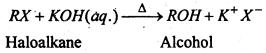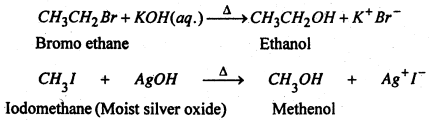(ii) Substitution of halogen by alkoxy group- Haloalkane when treated with sodium or potassium alkoxides form ethers.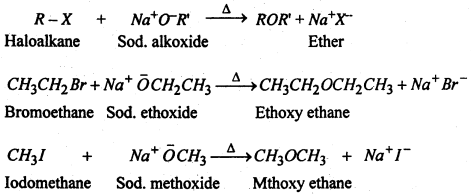(iii) Substitution of halogen by cyano group- Haloalkanes react with alcoholic solution of KCN to form alkane nitrile or Alkyl cyanides.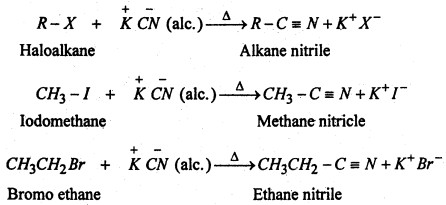(iv) Substitution of halogen by isocyanide group- When the aqueous ethanolic solution of a haloalkane is heated with silver cyanide, alkyl isocyanide or alkyl isonitricle is produced.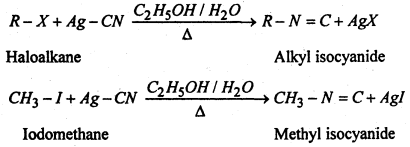(v) Substitution of halogen by nitre group- When an aqueous ethanolic solution of a haloalkane is heated with silver nitrite, nitroalkane is formed.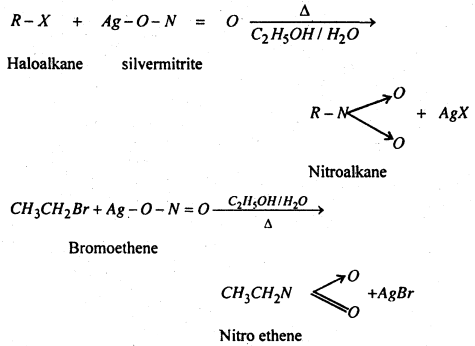(vi) Substitution of halogen by amino group- Haloalkanes when heated with an ethenolic solution of ammonia in a sealed tube at 373 K, from mixture of 1°, 2° and 3° amines along with quartenary ammonium salts.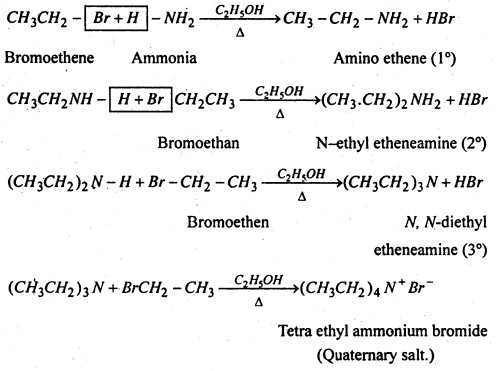(vii) Substitution of halogen by carboxylate group- Haloalkanes form esters when hated with an ethenolic solution of silver salt of a fatty acid.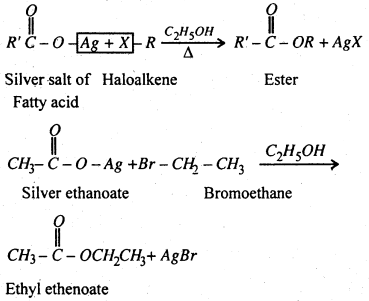Question 2.
Discuss the trends in chemical reactivity of group 15 elements.
Nitrogendiffers from other members of the family in its chemical reactivity. Some important trends in the chemical reactivity of this family are as follows.:
(a) Hydrides- All the members of group 15 form volatile hydrides of the formula MH3 where M = N, P, As, Sb and Bi. The lighter elements also form hydrides of the formula M2H4. Only nitrogen forms N3H.

(b) Oxides- Nitrogen has strong tendency to form Pπ-pπ multiple bonding with nitrogen and oxygen atoms whereas other elements of this group do not. Actually N forms five oxides with oxidation states ranging from + 1 + to +5 (N2O, NO, N2O2, NO2, N2O5) while other elements form oxides only in +3 and +5 oxidation states eq. P2O2, P2O5, Sb2O3, Bi2O2 etc.

(c) Halides- The elements of group 15 form halides of two types- trihalides MX3and pentahalides MX3 where M = N, P, As, Sb and Bi and X = F, Cl, Br and iodine. Nitrogen does not form pentahalides because of the absence of third shell in it.

Question 3.
Primary alkyl halide C4H9Br (A) reacted with alcoholic KOH to give compound (B). Compound (B) reacted with HBr to give compound (C) Which is an isomer of (A). When (A) was reacted with sodium metal it gave a compounds (D) C8H18 which is different from the compound when b-butyl bromide was reacted with sodium metal. Give the structural formula of (A) and write the equation for all the reactions.
C4H9Br has two primary alkyl halides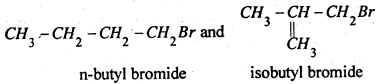n-Butyl bromide gives n-octane C8H18 which is different from the compound given by isobutyl bromide.
CH3·CH2·CH2·CH2·Br + Na + Br·CH2·CH2·CH2·CH3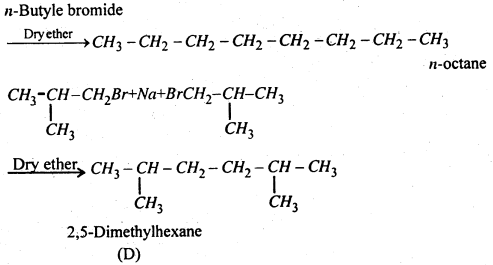Therefore (A) is isobutyl bromide which is confirmed from the following reactions: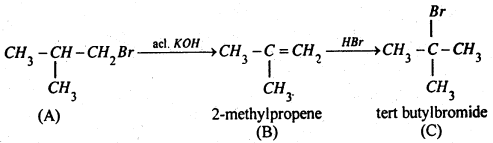Compound (C) is an isomer of compound (A). Hence compound (A) is isobutyl bromide.

Question 4.
(a) What is meant by coordination number of an atom in a crystal?
(b) What is the coordination number of atoms : (i) in a cubic close packed structure, (ii) in a body centred cubic structure.
(a) The coordination number of an atom in a crystal is the number of atoms which are the immediate neighbours of that atom in the crystal. In ionic crystals, coordination number of an ion in the crystal is the number of oppositely charged ions surrounding that particular ion.

(b) (i) The coordination number of atoms in a cubic close packed structure is 12 as it is touching 6 atoms in its own plane, 3 just above it and 3 just below it.
(iii) the coordination number of an atoms in a body centred cubic structure is 8 as the atom in the centre of the body of the unit cell is surrounded by 8 atoms placed at the 8 comers of the cube.

Question 5.
Give reactions of ammonia with the following : (a) Chlorine, (b) Magnesium (c) Bleaching powder, (d) Mercurous nitrate (e) Carbonyl chloride, (f) HCl, (g) CuSO4 solution, (h) Carbon dioxide, (i) Sodium.
(a) Reaction with Chlorine- When excess of ammonia is treated with chloride nitrogen is formed.
8NH3 + 3Cl2 → N2 + 6NH4Cl

(b) Reaction with magnesium- When magnesium is ignited in ammonia gas, magnesium nitride is formed with evolution of hydrogen gas
3Mg + 2NH3 → Mg3N2 + 3H2

(c) Reaction with bleaching powder- When a concentrated solution of ammonia is dropped overheated bleaching powder, nitrogen is evolved.
3Ca(OCl)Cl + 2NH2 → 3CaCl2 + 3H2O + N2

(d) Reaction with mercurous nitrate- Ammonia reacts with mercurous nitrate solution yielding a black precipitate which is a mixture of metallic mercury and nitrate of Millon’s base.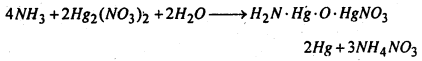(e) Reaction with carbonyl chloride- Ammonia reacts with carbonyl chloride giving urea.
2NH3 + COCl2 → NH2 · CO · NH2 + 2HCl

(f) Reaction with HCl- Being basic in nature ammonia reacts with hydrochloric acid and other acids forming ammonium salts.
NH3 + HCl · NH4Cl
NH3 + HNO3 · NH4NO3
2NH3 + H2SO4 · (NH4)2SO4

(g) Reaction with CuSO4 Solution- When an aqueous solution of ammonia is poured into an aqueous solution of CuSO4, cuppric hydroxide is precipitated which dissolves in excess of ammonia forming a soluble complex tetraammine copper (II) sulphate due to which the solution becomes blue.
NH3 + H2O → NH4OH
CuSO4 + 2NH4OH → Cu(OH)2 + (NH4)2 SO4
Cu(OH)2 + (NH4)2SO4 + 2NH4OH → [Cu(NH3)4]SO4 + 4H2O

(h) Reaction with carbon dioxide- When ammonia is heated with CO2 at high pressure an organic compound urea is formed.
2NH3 + CO2 → NH2 · CO · NH2 + H2O

(i) Reaction with sodium- When ammonia is passed over heated sodium, sodamide is formed.
2Na + 2NH3 → 2NaNH2 + H2
Liquid ammonia dissolves sodium forming a deep blue solution.

Question 6.
How is SO2 art air pollutant?
(i) SO2 is strongly irritating to the respiratory tract. SO2 at a concentration of 5 ppm causes throat and eye irritation resulting in cough, tears and redness in eyes. It causes breathlessness and affects larynx, i.e; voice box.

(ii) Even at a low concentration of 0.03 ppm, it has a damaging effect on plants. If exposed for a long time, it slows down the formation of chlorophyll resulting in injury to the leave including loss of green colour. This is called chlorosis.

(iii) SO2 dissolves in moisture present in air to form H2SO3, which damages building material especially marble.
CaCO3 + H2SO3 → CaSO3 + H2O + CO2

Question 7.
Why are halogens considered strong oxidising agents?
Due to low bond dissociation enthalpy, high electronegativity and large negative electron gas enthalpy, halogens have a strong tendency to accept electrons and thus get reduced.
X + e → X
Thus, halogens act as strong oxidising agents. Their oxidising power, however, decreases for F2 to I2.

Question 8.
Explain why inspite of nearly the same electronegativity, oxygen forms hydrogen bomb while chlorine does not.
Although O and Cl have about the same electronegativity, yet their atomic size (covalent radii much different : O = 66 pm and Cl = 99 pm. Thus, electron density per unit volume on oxygen is much higher than that on chlorine atom. Hence, oxygen forms hydrogen bonds while chins does not though both have approx, the same electronegativity.

Question 9.
Can PCl5 act as on oxidising as well as reducing agent? Justify.
The oxidation state of P in PCl5 is +5. As P has five electrons in its valence shell, it cannot increase its oxidation state beyond +5 by donating number from +5 to +3 or some lower value, So PCl5 acts as an oxidising agent. For example, it oxidises Ag to AgCl, Sn to SnCl4.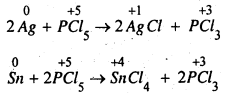Question 10.
Justify the placement of O, S, Se, Te and Po in the same group of the periodic table in terms of electronic configuration, oxidation state and hydride formation.
(i) Electronic configuration :
8O = [He] 2s2 2p4; 16S = [Ne] 3s2 3p4; 34Se = [Ar] 3d10 4s2 4p4
52Te = [Kr]4d105s25p4
and 84Po = [Xe]4f14 5d10 6s2 6p4
All these elements have same ns2 np4 (n = 2 to 6) valence shell electronic configuration and hence are justified to be placed in group 16 of the periodic table.

(ii) Oxidation states : They need two more electrons to form dinegative ions by acquiring the nearest noble gas configuration. So, the minimum oxidation state of these elements should be -2. Oxygen predominantly and sulphur to some extent being electronegative show and oxidation state of -2. Since these elements have six electrons in the valence shell, therefore at the maximum they can show an oxidation state +6. Other positive oxidation states shown by these elements are +2 and +4. Although, oxygen due to the absence of d-orbitals does not show oxidation states of +4 and +6. Thus, on the basis of minimum and maximum oxidation states, these elements are justified to be placed in the same group i.e., group 16 of the periodic table.

(iii) Formation of hydrides : All the elements complete their respective octets by sharing two of their valence electrons with 15-orbital of hydrogen to form hydrides of the general formula EH2 i.e., H2O, H2S, H2Se, H2 Te and H2Po: Therefore, on the basis of formation of hydrides of the general formula, EH2, these elements are justified to be placed in group 16 of the periodic table.

Question 11.
Why is dioxygen a gas but sulphur a solid?
Oxygen forms pπ – pπ multiple bonds. Due to small size and high electronegativity oxygen exists as diatomic (O2) molecules. These molecules are held together by weak van der Waals’ forces. Hence O2 is a gas at room temperature.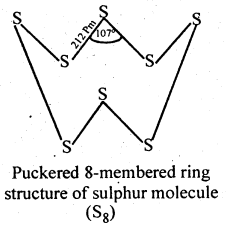Sulphur because of its bigger size and lower electronegativity, prefers to form S-S single bonds. Further because of stronger S-S than O-O single bonds, sulphur has a much greater tendency for catenation than oxygen. Thus, sulphur because of its higher tendency for catenation and lower tendency for pπ – pπ multiple bonds, forms octaatomic (S8) molecules having eight-membered puckered ring structure. Because of bigger size, the forces of attraction holding the S8 molecules together are much stronger. Hence, sulphur is a solid at room temperature.

Question 12.
Write main differences between the properties of white phosphorus and red phosphorus.
The difference in properties of white and red phosphorus is given in following table :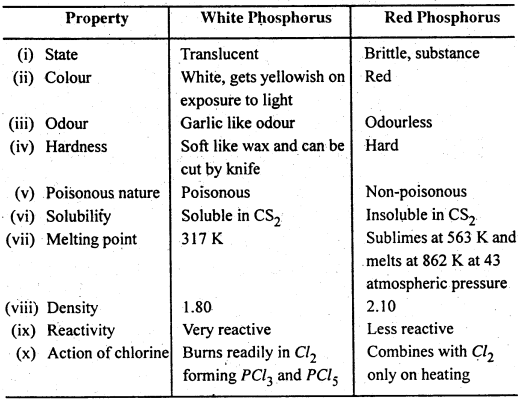Structure of white and red phosphorus are given below: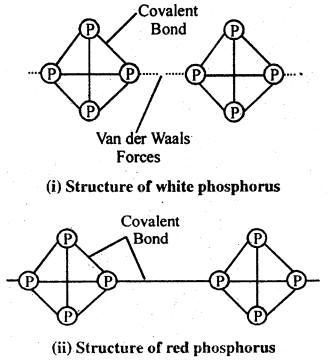Question 13.
(A) What do you mean by molecularity and order of reaction? What is relation between molecularity and order of a reaction?
(B) What do you mean by rate of reaction? Derive an expression for the rate constant of a first order reaction. What are the characteristics of a first order reaction?
(A) Molecularity of a reaction :
In a reaction A + 2B → C + D, one molecule of A reacts with two molecules of B to give on molecule each to C and D. The total number of molecules of A and B, in this reaction is called the molecularity of this reaction.

Thus the molecularity of reaction is the sum of number of a molecules of various reactants that take part in a chemical reaction as represented by a balanced chemical reaction.

The molecularity of the above reaction is three as one molecules of A and two molecules of B take part in the reaction.

The reaction in which only one molecules of the reactant takes part is called unimoleular reaction. For example, conversion of maleic acid into fumaric acid is a unimolecular reaction.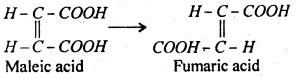The reaction in which two molecules of reactant take part is known as bimolecular reaction, for example, hydrolysis of ester is bimolecular because one molecule each of ester and water take part in the reaction.
CH3COOC2H5 + H2O → CH3COOH + C2 H5OH
The reaction in which three molecules of reactants are involved are called trimolecular reaction examples are
2NO + O2 → 2NO2
2FeCl3 + SnCl2 → 2FeCl3 + SnCl4
In the above examples three molecules take part in the reaction.
Hence the molecularity of these reactions are three.

Order of reaction-
It is not necessary that the concentrations of all the reactants take part in a reaction can determine the rate of reaction. Therefore, a new term “order of reaction” has been introduced.

The order of a reaction is defined as follows : “The order of a reaction in given by the number of atoms or molecules whose concentration changed during the chemical change ”

This is also defined as follows :
“The order of reaction given by the total number of atoms of molecules whose concentrations determine the velocity of the reaction.”
The rate equation of a general reaction mA + nB → pC + qD is expressed as $$\frac{d x}{d x}$$ = K[A]m n where m and n are small numbers (whole of fractional).

The order of reaction refers to the powers m and n in the rate of equation. The order with respect to A is m and with respect to B is n. Thus the order of reaction with respect to whole reactants is m + n. The value of m + n may be zero, whole number of or even fractional.

When the order of reaction is zero, it is called zero order reaction. For example, decomposition of ammonia in presence of M0 or W is a zero order reaction.
$$2 \mathrm{NH}_{3} \stackrel{\mathrm{M}_{0}}{\longrightarrow} \mathrm{N}_{2}+3 \mathrm{H}_{2}$$
Photochemical combination between H2 and Cl2 is also a zero order reaction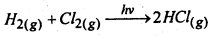The reaction whose rate of reaction is determined by one concentration term only, the order of the reaction is only and is known as first order reaction. For example, hydrolysis of ester by water in presence of mineral acid is a first order reaction.
CH3COOC2H5 + H2O → CH3COOH + C2H5OH

During hydrolysis the concentration of only ethyl acetate changes since water is present in large excess. Hence this is a first order reaction.

When the rate of a reaction depends upon two concentration terms, the reaction is known as a record order reaction. For example, hydrolysis of an ester by an alkali is a second order reation because during the course of reaction concentrations of both ester and alkali change.
CH3COOC2H5 + NaOH → CH3COONa + C2H5OH
Thermal decomposition of acetaldehyde is an example of second-order reaction.
2CH3CHO → 2CH4 + 2CO

A third order reaction is that those rate depends upon three concentration terms. Reduction of ferric chloride by stannous chloride studied by Noyes, is an examples of third order reaction.
2FeCl3 + SnCl2 → 2FeCl2 + SnCl4
The reaction between nitric oxide and hydrogen is also a third order reaction.
2NO + H2 → N2 + H2O2

Relation between molecularity and order of a reaction :-
Till recently, molecularity and order of reaction were regarded as synonymous. But it has now been found that in certain cases the two are not identical. For example, in inversion of cane sugar into glucose and fructose or in the hydrolysis is of ethylacetate in presence of acid catalyst apparently two molecules take part, making the reactions bimolecular. But actually the concentration only one of the reactants changes i.e. concentration of water does not appreciable, change as it is present in large excess.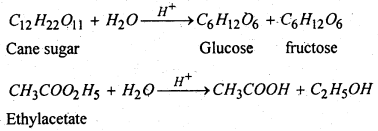Though, molecularity of a reaction often concides with its order,but the two need not be always identical.

The molecularity must be integral but the order of reaction may be zero or fractional. Whereas molecularity can be given on the basis of some proposed theoretical mechanism as to satify the experimental findings, the order of reaction can be obtained from experimental results.

(B) Rate of reaction- We know that the rate of a chemical reaction is directly proportional to the product of the active mass of the reactants. When the reaction proceeds the reactants are consumer and products are formed. Thus the concentration of reactants decreases and the concentration of products increases with time.

The rate can be determined by following the concentration of any of the reactants or products with time. The rate may be defined by the equation Average rate of reaction.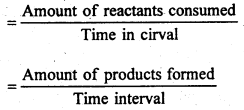The rate of a reaction is defined as the rate of change of concentration of either reactant or product per unit time.
Let us consider a simple reaction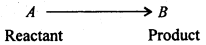It CA represents the concentration of the reactant A at time t, then the rate of the reaction may be defined as :
Rate = $$\frac{d\left(C_{A}\right)}{d t}$$
The minus sign in the above equation occurs because the concentration of the reactant decreases with increasing time.
If ‘a’ represents the initial concentration of A and x represents the concentration of product at time ‘t’, then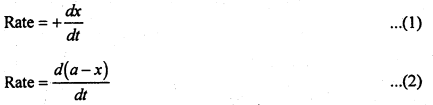In equation (1) sign is positive because concentration of the product increases with time. In equation (2) sign is negative since the concentration of reactant decreases with time.
Hence Rate = $$+\frac{d x}{d t}=-\frac{d(a-x)}{d t}$$